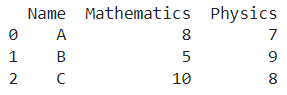# Convert Pandas DataFrame To NumPy Array

A DataFrame is the primary data structure of the Pandas library in Python and is commonly used for storing and working with tabular data.

A common operation that could be performed on such data is to convert a Pandas DataFrame to a numpy array in order to have the information in a more workable format.

To start working with Pandas, we first need to import it:

``import pandas as pd``

Here, we’ll need the NumPy library as well. To start working with NumPy, we first need to import it:

``import numpy as np``

## Running Example

Let us understand this operation with the help of an example. Consider the following DataFrame containing 3 students with names A, B, and C and their corresponding marks (out of 10) for two subjects, Mathematics and Physics.Code snippet for generating the above DataFrame:

``````import pandas as pd

# Dictionary for our data
data = {'Name' : ['A', 'B', 'C'], 'Mathematics' : [8, 5, 10], 'Physics' : [7, 9, 8]}

# DataFrame for the dictionary
df = pd.DataFrame(data)

# Printing the DataFrame
print(df)``````

Here, data is a dictionary we created to initialize the DataFrame. For this, we use the .DataFrame() function of the Pandas library which takes the dictionary as an argument and returns the required DataFrame.

Now, let’s say we need to convert this DataFrame into a numpy array to maybe have the information in a more tractable format. The resulting output would look like this :

[['A' 8 7]

['B' 5 9]

['C' 10 8]]

Let us look at different ways of performing this operation on a given DataFrame :

## 1. Convert Pandas DataFrame To NumPy Array

In this method, we use the DataFrame.to_numpy() function to convert the given DataFrame into a desired form, as a numpy array. The resultant NumPy array is obtained as the returned object.

The updates cannot be done in an in place manner therefore reassignment is required.  Let us take a look at the corresponding code snippet and generated output for this method:

``````# Importing required libraries
import pandas as pd

# Dictionary for our data
data = {'Name' : ['A', 'B', 'C'], 'Mathematics' : [8, 5, 10], 'Physics' : [7, 9, 8]}

# DataFrame for the dictionary
df = pd.DataFrame(data=data)

# Performing the operation
df = df.to_numpy()

# Printing the result
print(df)``````

Output :

[['A' 8 7]

['B' 5 9]

['C' 10 8]]

By default, the changes are made by reference so any changes you make in the returned array would be reflected in the original DataFrame as well. If you want the function to return a copy instead then we need to pass the parameter copy as True. Let us take a look at the corresponding code snippet and generated output for this method:

``````# Importing required libraries
import pandas as pd

# Dictionary for our data
data = {'Name' : ['A', 'B', 'C'], 'Mathematics' : [8, 5, 10], 'Physics' : [7, 9, 8]}

# DataFrame for the dictionary
df = pd.DataFrame(data=data)

# Performing the operation
df = df.to_numpy(copy = True)

# Printing the result
print(df)``````

Output :

[['A' 8 7]

['B' 5 9]

['C' 10 8]]

## 2. Using the np.array() function

In this method, we use the np.array() function to convert the given DataFrame into the desired form, as a numpy array.

The resultant numpy array is obtained as the returned object. The DataFrame is passed as a parameter to this function.

Let us take a look at the corresponding code snippet and generated output for this method:

``````# Importing required libraries
import pandas as pd
import numpy as np

# Dictionary for our data
data = {'Name' : ['A', 'B', 'C'], 'Mathematics' : [8, 5, 10], 'Physics' : [7, 9, 8]}

# DataFrame for the dictionary
df = pd.DataFrame(data=data)

# Performing the operation
df = np.array(df)

# Printing the result
print(df)``````

Output :

[['A' 8 7]

['B' 5 9]

['C' 10 8]]

## 3. Using the np.asarray() function

In this method, we use the np.asarray() function to convert the given DataFrame into a desired form, as a numpy array. The resultant numpy array is obtained as the returned object. The DataFrame is passed as a parameter to this function. Let us take a look at the corresponding code snippet and generated output for this method :

``````# Importing required libraries
import pandas as pd
import numpy as np

# Dictionary for our data
data = {'Name' : ['A', 'B', 'C'], 'Mathematics' : [8, 5, 10], 'Physics' : [7, 9, 8]}

# DataFrame for the dictionary
df = pd.DataFrame(data=data)

# Performing the operation
df = np.asarray(df)

# Printing the result
print(df)``````

Output :

[['A' 8 7]

['B' 5 9]

['C' 10 8]]

## Conclusion

In this topic, we have learned to convert an existing Pandas DataFrame to a numpy array, following a running example of test scores of students in different subjects, thus giving us an intuition of how this concept could be applied in the real-world situations. Feel free to reach out to info.javaexercise@gmail.com in case of any suggestions.

Trending